# RS Aggarwal Solutions for Class 6 Chapter 21 Concept of Perimeter and Area Exercise 21C

Exercise 21C of chapter 21 provides the students with basic knowledge of finding an area for a given shape. The measurement of the region enclosed by a plane figure is called the area of the figure. RS Aggarwal Solutions contain step by step explanation for the problems as per the understanding ability of students. The students can get a clear idea about the concepts which are explained in the chapter and perform better in the exam. RS Aggarwal Solutions for Class 6, Chapter 21, Concept of Perimeter and Area Exercise 21C PDF are provided here.

## Download PDF of RS Aggarwal Solutions for Class 6 Chapter 21 Concept of Perimeter and Area Exercise 21C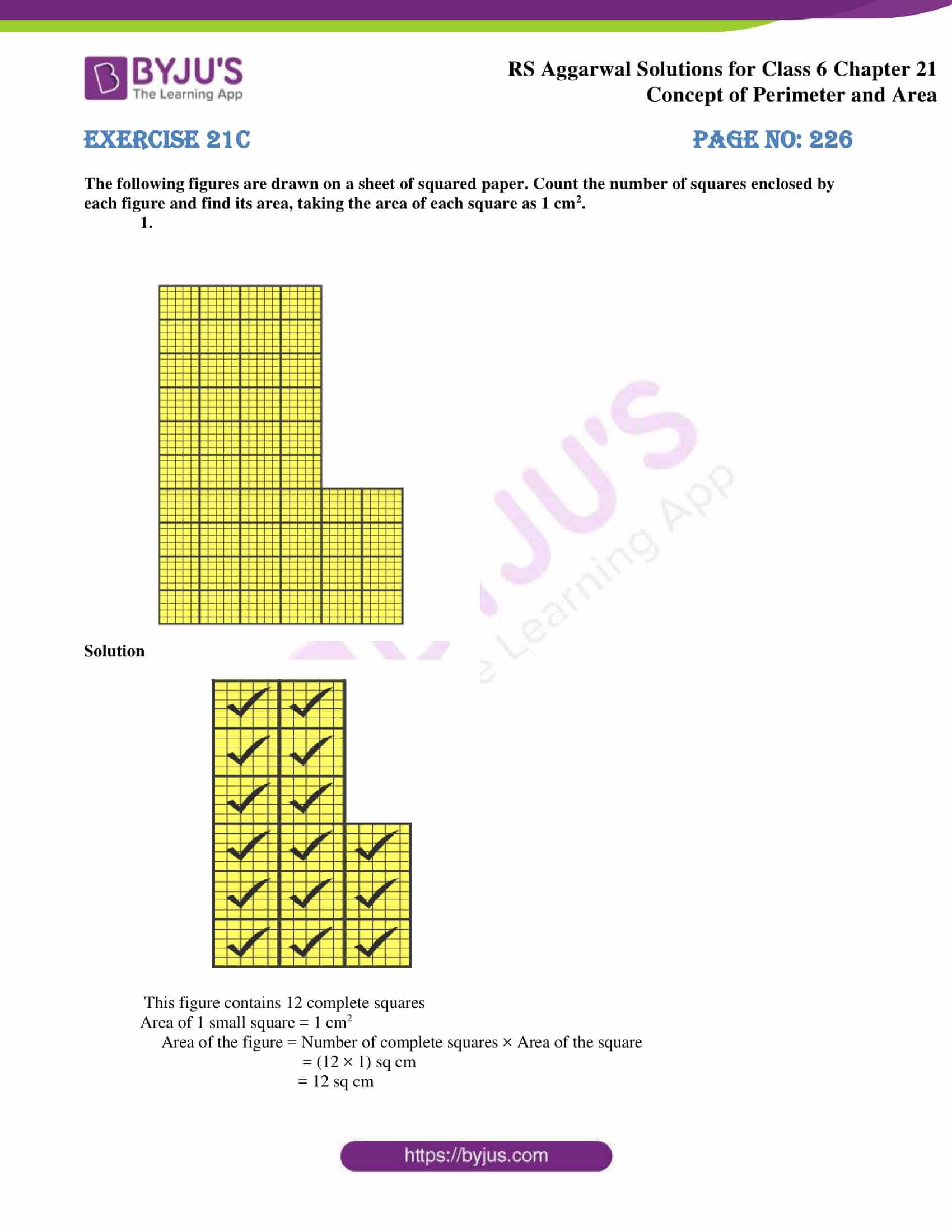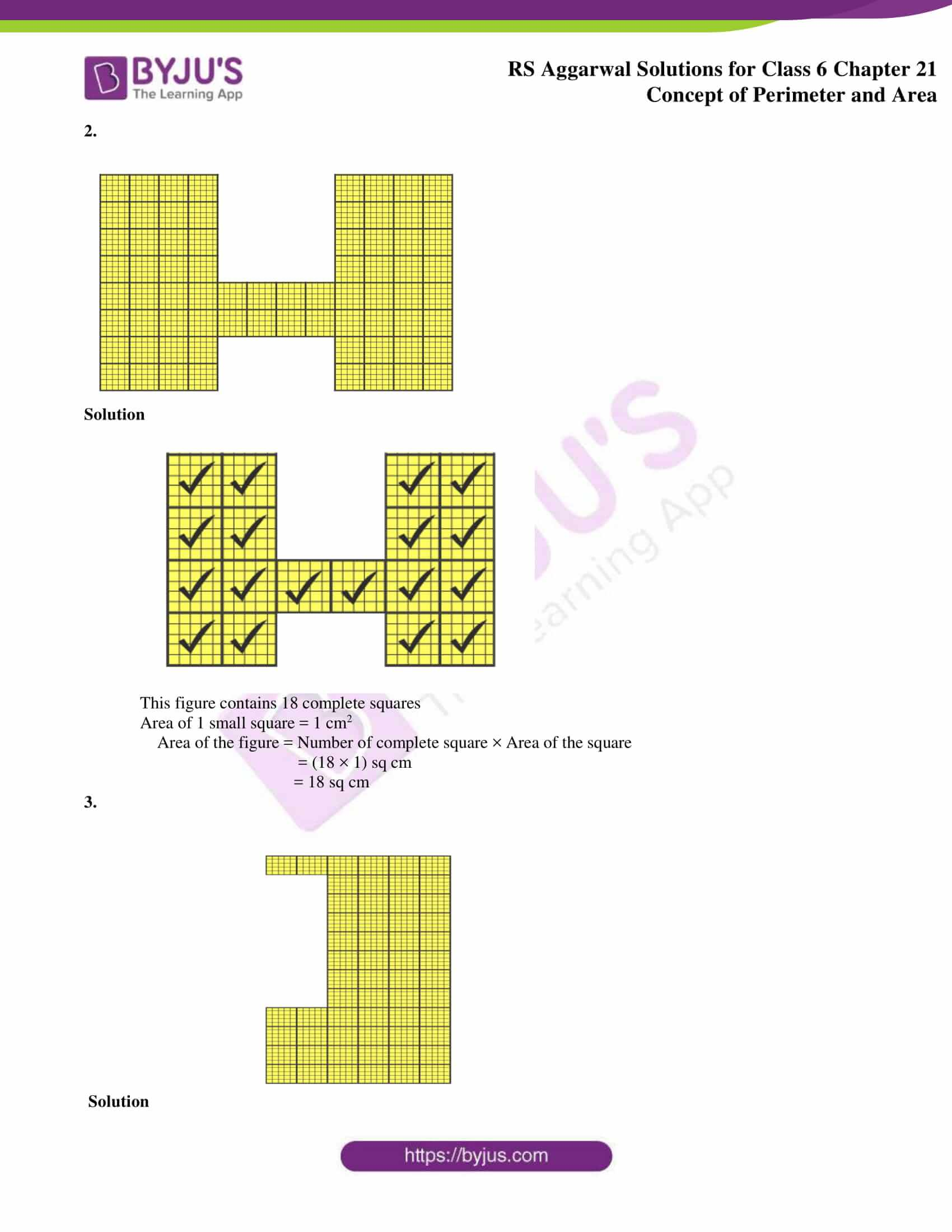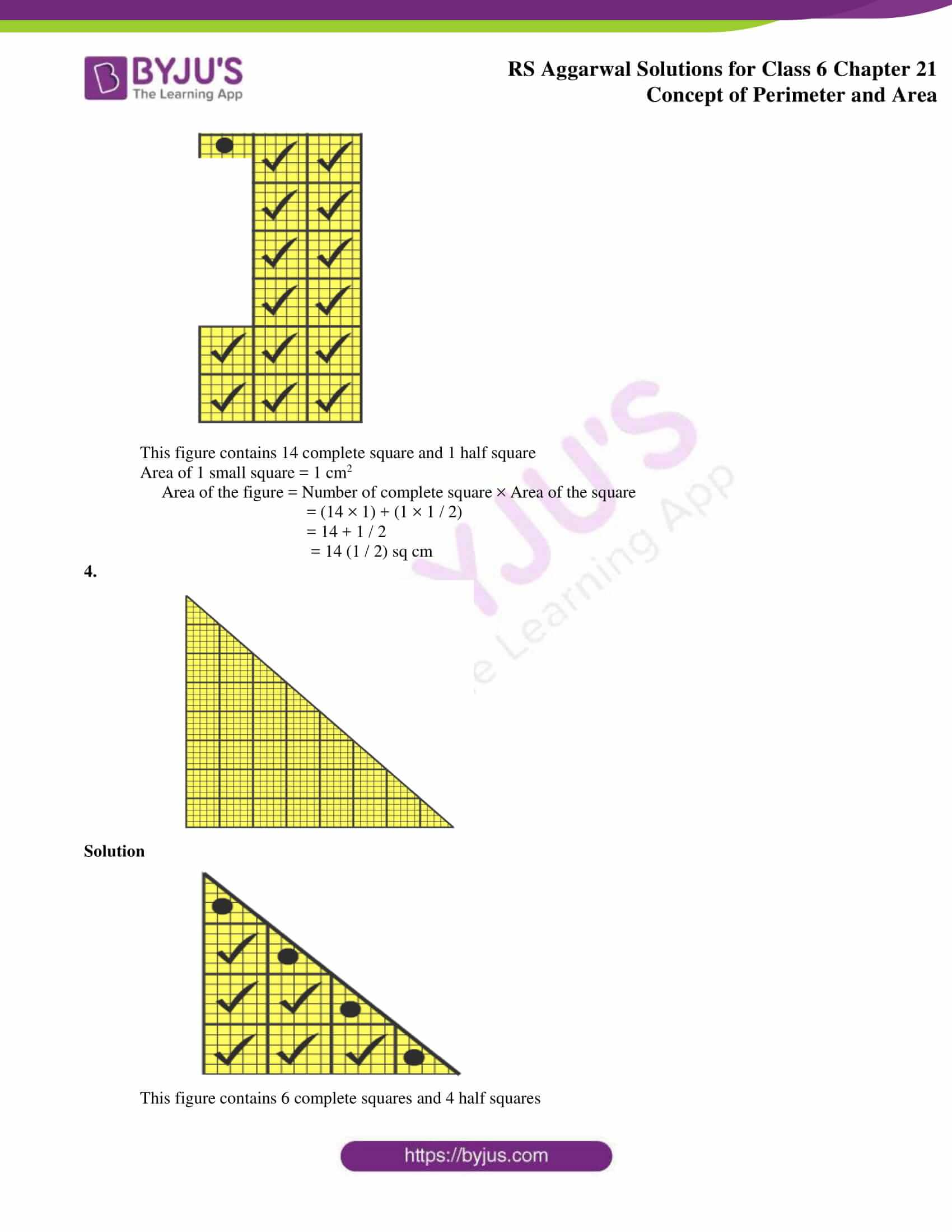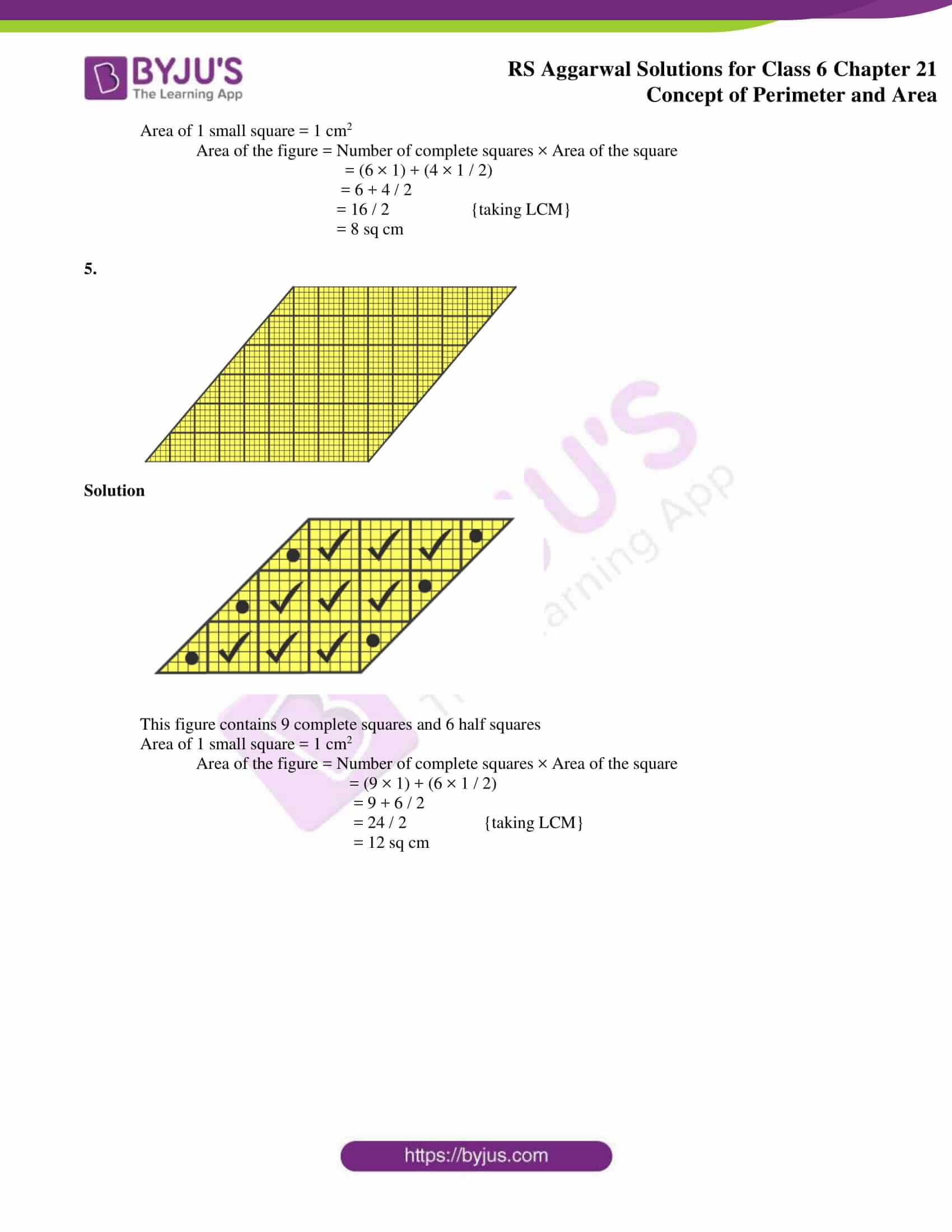### Access answers to Maths RS Aggarwal Solutions for Class 6 Chapter 21 Concept of Perimeter and Area Exercise 21C

The following figures are drawn on a sheet of squared paper. Count the number of squares enclosed by each figure and find its area, taking the area of each square as 1 cm2.

1.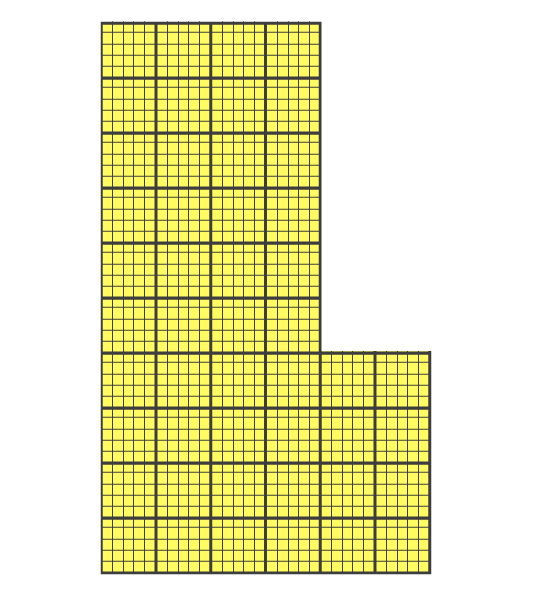Solution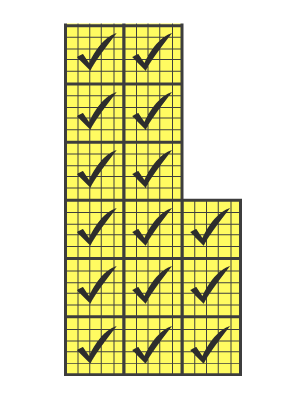This figure contains 12 complete squares

Area of 1 small square = 1 cm2

Area of the figure = Number of complete squares × Area of the square

= (12 × 1) sq cm

= 12 sq cm

2.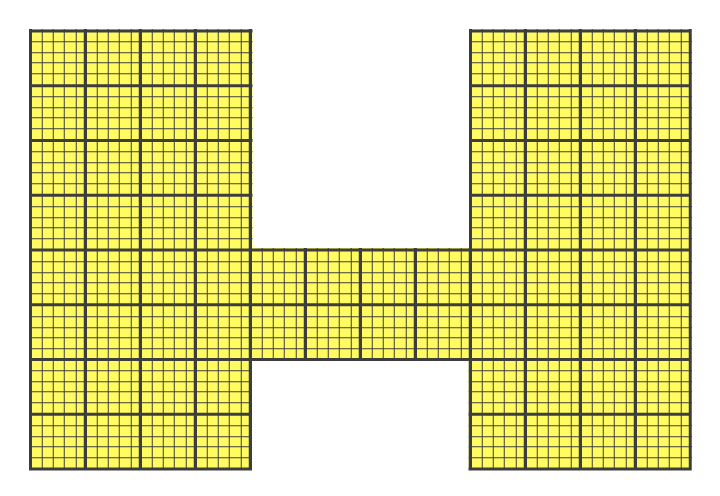Solution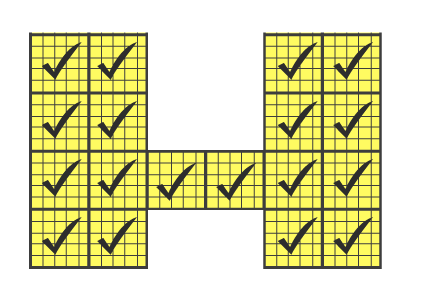This figure contains 18 complete squares

Area of 1 small square = 1 cm2

Area of the figure = Number of complete square × Area of the square

= (18 × 1) sq cm

= 18 sq cm

3.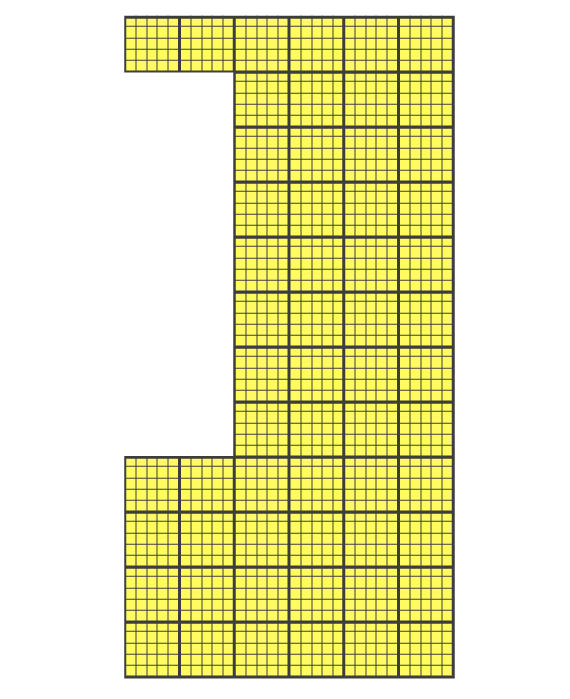Solution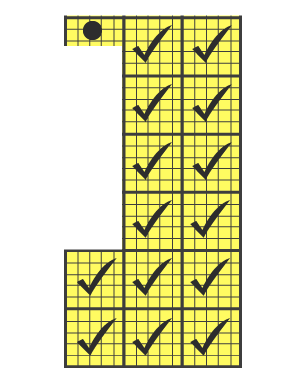This figure contains 14 complete square and 1 half square

Area of 1 small square = 1 cm2

Area of the figure = Number of complete square × Area of the square

= (14 × 1) + (1 × 1 / 2)

= 14 + 1 / 2

= 14 (1 / 2) sq cm

4.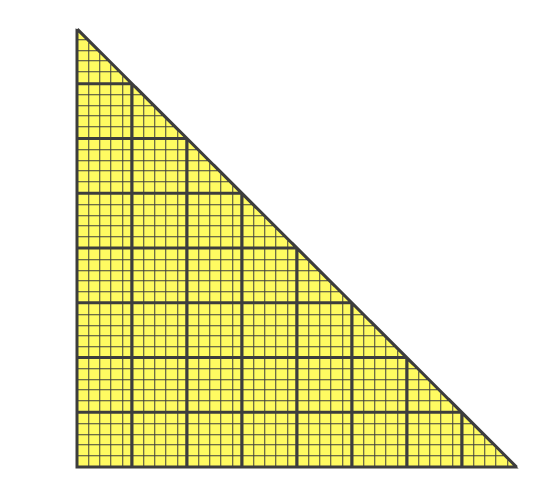Solution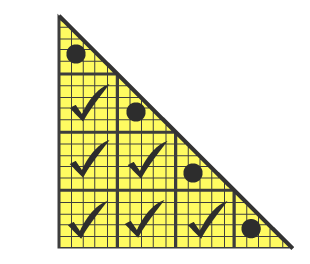This figure contains 6 complete squares and 4 half squares

Area of 1 small square = 1 cm2

Area of the figure = Number of complete squares × Area of the square

= (6 × 1) + (4 × 1 / 2)

= 6 + 4 / 2

= 16 / 2 {taking LCM}

= 8 sq cm

5.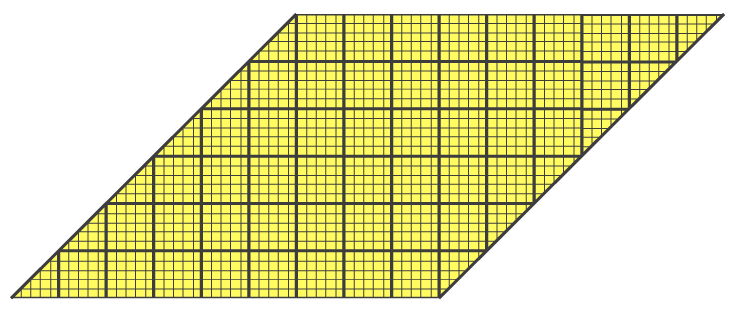Solution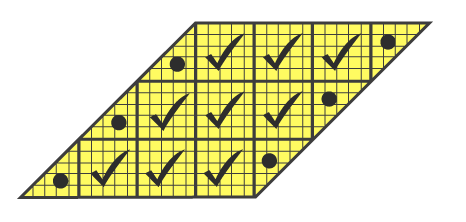This figure contains 9 complete squares and 6 half squares

Area of 1 small square = 1 cm2

Area of the figure = Number of complete squares × Area of the square

= (9 × 1) + (6 × 1 / 2)

= 9 + 6 / 2

= 24 / 2 {taking LCM}

= 12 sq cm

### Access other exercises of RS Aggarwal Solutions for Class 6 Chapter 21 Concept of Perimeter and Area

Exercise 21E Solutions